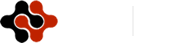International Journal of Chemical and Biomolecular Science, Vol. 1, No. 3, October 2015 Publish Date: Sep. 9, 2015 Pages: 197-210

Ab Inito Calculations of Methanol Clusters and Its Electrical Parameters

1Department of Chemistry, K. N. Toosi University of Technology (KNTU), Tehran, Iran

2Department of Chemistry, Payam Noor University, Tehran, Iran

3Drug Applied Research Center, Tabriz University of Medical Sciences, Tabriz, Iran

4Pharmaceutical Analysis Research Center, Tabriz University of Medical Sciences, Tabriz, Iran

5Department of Nanochemistry, Nano Technology Research Center and Faculty of Chemistry, Urmia University, Urmia, Iran

Abstract

Extensive ab inito calculations have been performed in HF, DFT and MP2 levels for several possible structures of methanol clusters (CH3OH)n, n = 1-6. The calculations of the structures, primary and secondary hydrogen bond properties, stabilization energy, vibrational frequencies and intensities of eighteen minima of methanol clusters have been presented perfectly. In this work, a systematic study for 18 possible structures of methanol clusters, from isolated molecule to six molecules in the gas phase. This work reports all of the stable geometry parameters, O-H stretching frequencies in clusters and denotes to distinguished molecules with intensities at any molecules, oxygen and hydrogen charge in clusters and primary and secondary hydrogen bond strength performed with the methods. Cyclic, chain and branched-cyclic are structural types that methanol clusters have been shown in these frameworks.

Keywords

Ethanol-Water Clusters, Secondary Hydrogen Bond, Electron Density, Hydrogen Bonds

Contents

1. Introduction

Ethanol clusters, groups of methanol held together by hydrogen bonds, have been the subject of a number of intense experimental and theoretical investigations because of their importance in understanding solution chemistry (Islam, Pethrick and Pugh, 1998), in the industry of renewable fuels (Choi, et. al., 2004), and a large number of chemical and biochemical processes (Azizi, et. al., 2007). From a theoretical point of view, many different models of methanol clusters have been studied for understanding the characteristics of hydrogen bonds. A number of ab initio calculations have also been carried out to investigate the strength of the hydrogen bonds and their cooperativity but all of them have not been arrived in details of these bonds and its clusters. Hartree-Fock, Density Functional, Moller-Plesset Perturbation theories and Monte Carlo, simulations have been previously carried out on methanol clusters (Sum and Sandler, 2000, Boyd, S.L. and Boyd, R.J., 2007, Haughney, Ferrario, and McDonald, 1987, Handgraaf, Van Erp and Meijer, 2003, Morrone and Tuckerman, 2002).

In this work, a systematic study for 18 possible structures of methanol clusters, from isolated molecule to six molecules in the gas phase. This work reports all of the stable geometry parameters, O-H stretching frequencies in clusters and denotes to distinguished molecules with intensities at any molecules, oxygen and hydrogen charge in clusters and primary and secondary hydrogen bond strength performed with the methods which few investigations (Mejia, Espinal and Mondragon, 2009) are showing this effect.

2. Computational Details

In this work all of the structures optimized by the polarized basis set, 6-31G (d) in DFT, MP2 and HF methods. The selection of the computational approaches was based on three criteria. First, DFT is showed in many investigations to be useful for describing hydrogen bonded systems (Boyd, S.L. and Boyd, R.J., 2007). Secondly, MP2 is used according to the recommendation by A. Garcia-Muruais and et al., (Garcia-Muruais, 2000). Thirdly, HF method was selected in order to compare with earlier methods. The Basis set (6-31G(d)) was selected according to the recommendation by A. Garcia-Muruais and et.al., (Garcia-Muruais, 2000), too. All of calculations are carried out with the Gaussian 03 software package (Frisch, 1998). B3LYP is selected as DFT method. To facilitate the representation of different combinations of same size structures, the IUPAC naming rule will be used in spite of different that methanol molecules will be substituted carbon atoms and digits are engraved on oxygen atoms. In this study, we will be investigated methanol clusters from one to six molecules with following details: 1 - Expansive conformers particularly for hexamer cluster, 2 - Identification of primary and secondary hydrogen bond and its effects in stability of clusters, 3 - Oxygen and hydrogen atoms charges 4-  the distinguish vibrational frequencies between each molecules in clusters and its intensities, the fact that these details were not found in previous calculations. The average energy of a single methanol molecule in a methanol cluster can be calculated as

E^n,^average = E^n/n                             (1)

The Stabilization energy (SE in Kcal/mol) for each cluster is obtained as showe below.

SE = E^cluster – n*E^monomer                  (2)

Ecluster: Total energy of cluster, Emonomer: Energy of a single methanol molecule, n: number of monomers.

The stabilization energy per hydrogen bond (SE/nH) and delta’s SE (delta SE) for each cluster is obtained, in order to indicate change in strength of hydrogen bond with increasing cluster size.

The counterpoise procedure was used to correct the stabilization energy for the basis set superposition error (BSSE) (Boys and Berardi, 1970). The AIM2000 package (Bader, Biegler-Konig and Schonbohm, 2002) was applied to obtain the nature of hydrogen bonding interactions, the characteristics of the hydrogen bond critical points (BCP) appearing in the studied clusters. The total electron density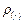is calculated from the wave function of the equilibrium geometry of the various clusters at B3LYP/6-31G(d) level.

3. Results and Discussion

3.1. Cluster Geometry

Figure S1(was showed in supplementary data) expressions the schematic representation of the stable geometry for (CH3OH)n, n =1-4, along with the number of hydrogen bonds (nH) and total dipole moment values in Debye as obtained from DFT calculations. The values in parentheses and brackets correspond to the μ values obtained from MP2 and HF calculation respectively. The dipole moment value in experimental liquid phase molecule is 1.7 D (Garcia-Muruais, 2000). The methanol dimmer, the smallest methanol cluster which constitutes the fundamental step in the study of methanol clusters, has been studied in great detail experimentally (Lovas, 1995) as well as theoretically (Odutola and Dyke, 1980). According to the figure S1 and table S1 (was showed in supplementary data), it has been established that the most stable structure of a methanol dimmer is of the Cs symmetry and that it has a single hydrogen bond with strength of - 7.5, - 4.9 and - 5.5 kcal /mol at the DFT, MP2 and HF methods respectively.

The equilibrium geometry for the cyclic trimmer is flat, resulting in poor overlap of the sp3 orbital compared to the probable noncyclic structures. In such the structure, each monomer behaves as a donor as well as an acceptor.

To facilitate the representation of different combinations of same size structures, the IUPAC naming rule will be used in spite of different that methanol molecules will be substituted carbon atoms and digits are engraved on oxygen atoms. Tetramer clusters in figure S1 according to IUPAC naming rule, consist of:

1) 4-Chain: butane methanol

2) 1-M-CPr: 1- methanol cyclopropane methanol

3) 4-Cyclic: Cyclo butane methanol

All of the methods have established an almost harmonic cyclic structure with C1 symmetry to correspond to the global minimum for the tetramer. In these geometries, 4cyclic with the methyl agents periodically above and below the plane of the O-O-O-O ring is the most stable of all conformers. As a result, excessive rise was showed in the stabilization energy and excessive drop was indicated in the dipole moment, if we don’t take into account ZPE correction. If we include the ZPE corrections, the 1MCPr structure is found to be more stable than the 4 Cyclic by 6.6 kcal/mol at the DFT/6-31G(d) level (see table S1). The triangular ring in conformer of 1MCPr is like in structure to triangular ring in trimmer conformer excepting length of faces which in 1MCPr face of (1-3) decreased and faces of (1-2) and (2-3) in order to steric hindrance increased, that numbers within parentheses means the methanol numbers in interested clusters.

The pentamre isomers studied were the:

1) 5Chain: pentane methanol

2) 1-M2-CPr: 1-(methanol)2 cyclopropane methanol

3) 1-M-CBu: 1-methanol cyclobutane

4) 5Cyclic: cyclopentane methanol

According of the figure 1 and table S2, the above arrangement is in stabilization energies parameters including the ZPE and BSSE corrections for pentamer isomers. In the other word, pentagonal ring of methanol molecule, similar else clusters, is the most stable structure for the pentamer and can be resulted that hydrogen bonding length is the lowest. A detail of the hydrogen bonding length is given in table 1 and discussed later in the text.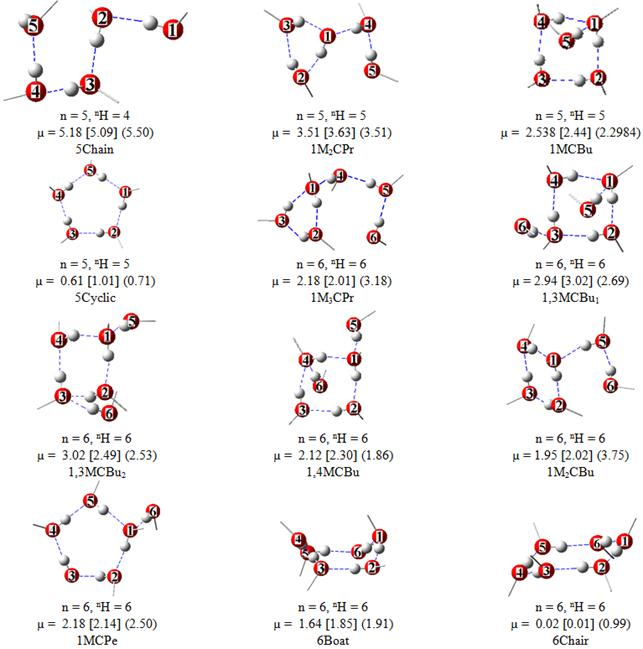Figure 1. Schematic representation of the most stable geometry for (CH3OH)n, n =1-4, along with the total stabilization energy (SE) values in kcal/mol, the number of hydrogen bonds (nH) and total dipole moment values in Debye as obtained from HF calculations using the 6-31G(d) basis set. The values in parentheses correspond to SE and µ values obtained from DFT calculations using B3LYP parameterization.

Table 1. Primary hydrogen bonding length, electron density (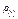) and Laplacian of electron density at BCP (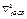) in methanol clusters from 5 to 6 molequels at the DFT/6-31G(d) method.is in negative values. All of data were calculatet in DFT level.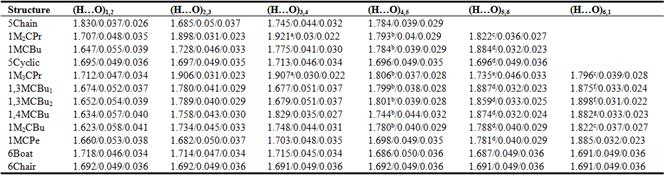a For Hydrogen Bonding between (H…O)3,1.

b For Hydrogen Bonding between (H…O)4,1.

c For Hydrogen Bonding between (H…O)6,5.

d For Hydrogen Bonding between (H…O)5,1.

e For Hydrogen Bonding between (H…O)5,4.

f For Hydrogen Bonding between (H…O)6,3.

g For Hydrogen Bonding between (H…O)6,4.

The pentagonal ring in 5Cyclic is no planar, with a structure that resembles an envelope. According to the above arrangement, in n = 5, with increasing ring's size stability of structure increased. Comparing of the average energy of a single methanol molecule in these clusters in third methods showed that average stabilization energy of a single methanol molecule in DFT calculations is -2.81 kcal/mol larger than HF calculations and -3.41 kcal/mol larger than MP2 calculations. The strongest hydrogen bond (1.647 Å) related to interaction between hydroxyl agents of the first molecule with oxygen atom of the second molecule in 1-M-CBu cluster (see table 1 and figure 1). Comparing of stabilization energy between 5Chain with 4Chain, 1-M2-CPr with 1-M-CPr and 5Cyclic with 4Cyclic will be show that increasing one chain methanol to structure will be cause to increasing stability in this set: cyclo butane structures > cyclo propane structures > chain structures.

The structure of (CH3OH)6 represents eight conformers with following SE arrangement:

1) 1-M3-CPr: 1-(methanol)3 cyclo propane methanol

2) 1, 3-M-CBu1: 1, 3-di methanol cyclo butane methanol (trans isomer)

3) 1, 3-M-CBu2: 1, 3-di methanol cyclo butane methanol (cis isomer)

4) 1, 4-M-CBu: 1, 4-di methanol cyclo butane methanol

5) 1-M2-CBu: 1-(methanol)2 cyclo butane methanol

6) 1-M-CPe: 1-methanol cyclo pentane methanol

7) 6Boat: cyclo hexane methanol (boat isomer)

8) 6Chair: cyclo hexane methanol (chair isomer)

Table S1 and table 2 were dictated that there are two large ring clusters in hexamer isomers that at HF and MP2 methods, 6Chair is the most stable cluster and at DFT method, 6chair in company with 6Boat are the most stable clusters. The mean value of H-O-H bond angles in Chair and Boat conformers at DFT method is 114.8º and 109.5º and comparing its between both ring clusters show that the Chair conformation is the most favored structure because there isn't angle strain since all bond angles remain in the beast position. Another difference is pattern of orientation of the methyl groups. In the Chair conformer the methyl groups are being above and below alternately with the ring plane. Though, in the Boat conformer they are being above below- in planar.

According of the table 2 and table S1, SE comparing of cis and trans isomers that is known as geometric isomerism, show that against the general cis and trans isomers 1,3-M-CBu2 (cis) is higher than 1,3-M-CBu1 (trans) albeit there is steric hindrance in cis isomer. The cause of this effect is the formation of three robust secondary hydrogen bond between O5…..HC4, O5…..HC6 and O6…..HC2 with bond length of 2.415, 2.572, 2.385 Å respectively (Ox and HCx are oxygen and methyl's hydrogen of X molecule) (see next section, Table 3). We effort for optimized 6chain have been jointed with failure and this structure has been exchanged to 1-M3-CPr. With an increase in the size of methanol clusters, the number of structural parameters also increased. Therefore, we have plotted the mean values <r>C-O (Figure 2a), <r>O-H (Figure 2b), <r>O…H (Figure 2c), COH (Figure 2d) and OHO (Figure 2e) for different values of n, in Figure 2. According to the Figure 2a, the C-O bond length at HF method in all of clusters have a constant amount and involve 1.40 (Å) values. Although, DFT and MP2 values consistently larger than the HF values however have not a constant imitation. The values of <r>O-H and <r>O…H are exactly on basis of hydrogen bonding existence conversely. Both parameters follows on a regular base, it means in all of clusters with equal molecules increasing of <r>O-H and decreasing of <r>O…H will be show in parts b and c of Figure 2 along with cluster stability. It is a next result that with arise of molecule number; the above consequence will be interrupt. However, it must be added that DFT and MP2 calculations tend to predict slightly stronger and shorter hydrogen bonds than those of HF. Figure 2d deals with the relation between the COH value and the cluster size for n=1-6 and its isomers. It is nearly a constant amount for three methods, emphasizing the fact that the ''structures" of the methanol molecules in clusters predicted by the each calculations are nearly the same and also in Figure 2e, the almost identical lines for anyone calculations, are emphasizing the fact that the "shapes" of the clusters predicted are nearly the same. With an increase in the size of the methanol clusters, there is an increase in stabilization energy (SE) values, as shown in Figure 3.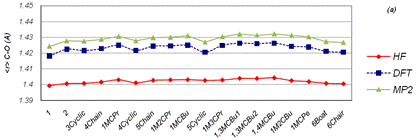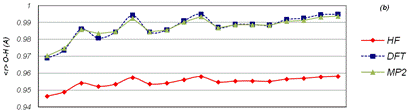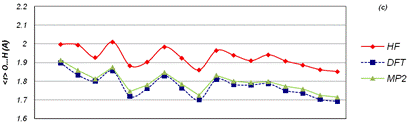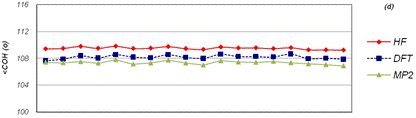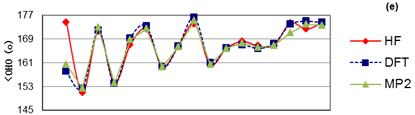Figure 2. Schematic representation of some of the different possible low-energy structures of (CH3OH)n, n=5-6, which is omitted methyl agent for the sake of clarity, along with the total stabilization energy (SE) values in kcal/mol, the number of primary hydrogen bonds (nH) and total dipole moment values in Debye as obtained from HF calculations using the 6-31G(d) basis set.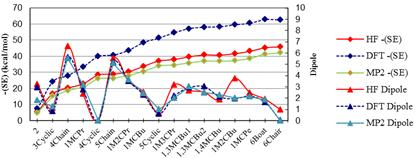Figure 3. Mean values of various geometrical parameters for the all of the conformers of (CH3OH)n, n=1-6, as obtained from HF calculation (solid line) and DFT calculations (dash line).

Table 2. Effect of BSSE and ZPE corrections on SE (kcal/mol) at the DFT/6-31G(d) level for (CH3OH)n , n=6.

 -SE Structure BSSE Uncorrected for BSSE and ZPE BSSE corrected ZPE corrected 1M3CPr -16.6 54.6 38.0 45.7 1,3MCBu1 -17.8 57.2 39.4 48.2 1,3MCBu2 -16.6 58.1 41.5 55.3 1,4MCBu -17.6 58.3 40.7 55.8 1M2CBu -16.5 59.6 43.0 50.7 1MCPe -15.6 60.5 45.0 51.8 6Boat -14.7 62.8 48.1 54.3 6Chair -12.8 62.5 49.6 54.4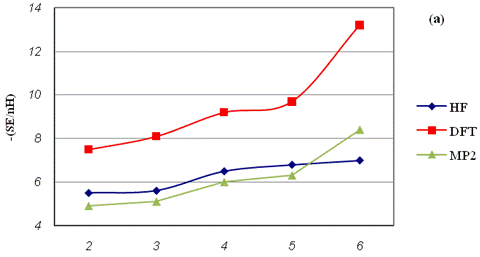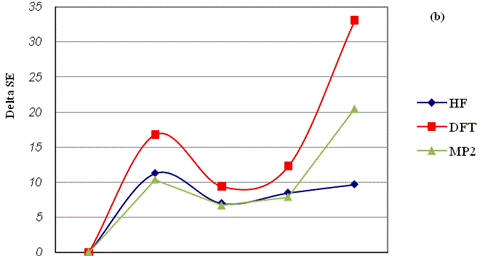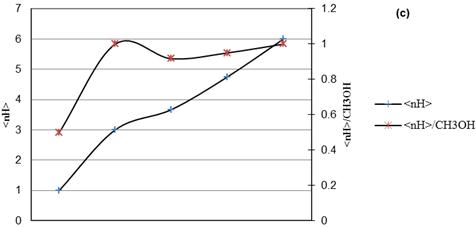Figure 4. Variation of the SE value and dipole value for the most stable geometry (CH3OH)n , n=2-6 as obtained from HF and DFT calculations

There is a little increase in the mean strength of the primary hydrogen bonds as n goes from 3 to 4 in HF calculations and there is noticeable increasing as n goes from 5 to 6 in other methods. In HF method, for n = 5 and 6, there is a very slight increase in the mean primary hydrogen bond strength. It becomes clear from the incremental values of absolute SE (i.e., the increase in absolute SE values with the increase in n by 1) plotted as a function of n in Figure 4b that there is an alternation in stability as n increases. The old n-mers have a slightly greater stability than the even n-mers. Methanol clusters with n = 3, 5 become particularly more stable. The average number of primary hydrogen bonds (<nH>/CH3OH) per methanol molecule increases up to n = 3 but then levels of around one as n approaches 6.

3.2. Atom in Molecules Analysis

In order to characterize the hydrogen bonding interaction between two methanol molecules, the electron density () at bond critical points was taken into account. According to AIM theory, any chemical bond including closed-shell interactions (e.g. hydrogen bonding) is characterized by the bond critical points (BCP). The BCP (3, -1) are characterized by a rank (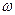) of 3 and a signature (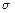) of -1. This means that the electron density at this point is a maximum in the plane defined by the corresponding eigen vectors but is a minimum along the third axis which is perpendicular to this plane. Although, in some of the studied clusters, ring critical points (RCP) and cubic critical points (CCP) were obtained.

In order to the same results in geometry parameters for DFT and MP2 levels, other theoretical calculations including AIM analysis and vibrational frequencies were performed only in DFT level. Tables 1, 3 and S3 shows the variation of the electron density (), Laplacian of electron density at BCP (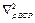), primary and secondary hydrogen bonding length of interested structures. Figure 5 gives a picture of how cluster size and different spatial arrangements impact electron density of a methanol cluster. The maximumvalues are observed in the cyclic structures even though from n=4 onwards they are uniformly around 0.048 a. u. .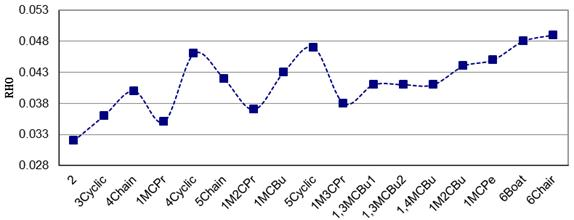Figure 5. Variation of  (a) Stabilization energy per hydrogen bond for (CH3OH)n, n = 2-6, corresponding to the most stable structure of the individual methanol cluster, (b) incremental stabilization energy (delta of SE) for n = 2-6, and (c) the number of primary hydrogen bonds (nH) and the average number of primary hydrogen bonds per methanol molecule (<nH> = nH/n) with an increase in n, as obtained from HF/6-31G(d) calculation. The values of  SE are reported in kcal/mol.

Interaction of the methyl's hydrogen of one molecule with oxygen's another molecule is called secondary hydrogen bond. Even though, the C-H…O interactions are weaker than the primary hydrogen bonds, that mentioned in earlier paragraph, they seem to influence the stability of some clusters and its vibrational frequencies that will be explained in next paragraph.

The first secondary hydrogen bonds have been seen in tetramer isomers. According table 3 in 1-M-CPr isomer there are two secondary hydrogen bonds that cause making of differences between triangular ring of this isomer and trimmer ring in 3 Cyclic structure. The first secondary hydrogen bonding with have 2.48 Å bond length is between Oxygen of fourth molecule (O4) and methyl's Hydrogen of the second molecule (HC2) by electron density of 0.011 a. u., and secondary is between Oxygen of the third molecule O3 and methyl's Hydrogen of fourth molecule HC4 (with bond length 2.70 Å and by electron density of 0.006 a. u.). Electrical parameters comparing of 3Cyclic and ring of 1-M-CPr show that existence of these secondary hydrogen bonding to be caused decreasing O1…O2 length in spite of that existence steric hindrance of methyl's group. Also, this effect to be caused that primary bonding between O2 and H1 is liner than other hydrogen bonds in ring of 1-M-CPr (see Table S4). The above results will be seen at 1-M2-CPr and at 1-M3-CPr isomers too. According to the table 3, in square rings the most SHB be found in 1, 3-M-CBu2 and 1-M-CBu with three SHB and 1, 3-M-CBu1 and 1, 4-M-CBu with four SHB number of its isomers. In addition to AIM results, the mulliken charge for HSB involving oxygen atoms which were presented in table S6, is the other evidence for exist several of secondary hydrogen bond in these isomers.

Table 3. Secondary hydrogen bonding length (SHB), electron density () and Laplacian of electron density at BCP () in methanol clusters at the DFT/6-31G(d) method.is in negative values. Calculation was obtained in DFT method.

 Structure SHB and its length SHB1 SHB2 SHB3 SHB4 Length/RHO/ Length/RHO/ Length/RHO/ Length/RHO/ 1 MCPr O4…HC2 O3…HC4 2.4770/0.011/0.008 2.703/0.006/0.005 1 M2CPr O5…HC2 O3…HC4 2.340/0.014/0.01 2.695/0.006/0.006 1 M3CPr O6…HC2 O6…HC4 O3…HC4 2.363/0.013/0.009 2.466/0.010/0.008 2.768/0.005/0.005 4 Chain O4…HC2 2.431/0.011/0.009 5 Chain O2…HC5 O1…HC3 2.354/0.013/0.01 2.506/0.01/0.008 1 MCBu O5…HC2 O5…HC3 O4…HC5 2.691/0.006/0.005 2.576/0.009/0.007 2.799/0.005/0.005 1 M2CBu O6…HC1 O6…HC2 2.501/0.009/0.008 2.473/0.010/0.008 1,3 MCBu1 O5…HC3 O5…HC2 O6…HC4 O4…HC5 2.537/0.009/0.008 2.628/0.006/0.006 2.52/0.010/0.008 2.816/0.005/0.004 1,3 MCBu2 O5…HC4 O5…HC6 O6…HC2 2.415/0.011/0.009 2.572/0.009/0.007 2.385/0.013/0.009 1,4 MCBu O5…HC4 O5…HC6 O6…HC2 O6…HC3 2.570/0.008/0.007 2.586/0.008/0.007 2.407/0.012/0.009 2.465/0.010/0.008 1 MCPe O6…HC5 O4…HC6 2.436/0.012/0.009 2.676/0.006/0.005 6 Boat O4…HC1 2.555/0.009/0.007

3.3. Vibrational Frequency of Isomers

Frequency calculations have been carried out at the B3LYP/6-31g(d) level. The scaling of the frequencies has been done with a scaling factor of 0.9613 for DFT level as recommended by the Gaussian 03 package. Tables 4 and S7 list the scaled frequencies (cm-1) with all of the intensities for these clusters along with the frequency of a single molecule and intensity of molecule’s vibration (IMV in Tables 4 and S). According to these tables secondary hydrogen bonds lead to decreasing of intensities for inclusive oxygen atoms vibration which this effect have been seen in 1,3MCBu1 and 1,3MCBu2 very clearly. For instance, in 1,3MCBu1 isomer in order to exist four SHB containing O4, O5 and O6, will be see the least effect in stretching frequencies of these oxygen atoms in table 4.

Table 4. Stretching frequencies calculated at HF/6-31G(d) optimized geometry for O-H in (CH3OH)n, n = 5,6 and its isomers. Frequencies are unscaled in cm-1 and IMV (intensity of molecule’s vibration) denotes to O-H intensities at any molecule.

 Structure Frequency Intensity IMV Structure Frequency Intensity IMV 5Chain 3614 27.3 5 1,3MCBu2 3486 502.1 5>>1=4=6 3384 661.8 1>>2 3457 541.8 6>>2=3=5 3356 732.7 3=4 3344 707.9 2>>1=3=6>4 3279 843.5 3>>2=4 3332 624.5 4>>1=3=5 3132 1061.7 2>>3>1=4 3113 2107.1 1>>3>2 3018* 334.8 3>>1=4>2=5 1M2CPr 3444 568.0 3>>1=2=4>5 1,4MCBu 3476 471.7 5>>1=3=4=6 3400 268.3 2=4=5>1=3 3466 574.6 6>>3>4=5 3392 625.1 2>3=5>>1=4 3405 465.6 3>>6>2=4 3339 806.5 5>>4>1=2=3 3261 1354.2 4>>2>1=3=5=6 3097 757.4 1>>2=3=4=5 3235 517.0 2>>1=3=4>5 2966* 962.3 1>>2=3=4=5 1MCBu 3466 459.4 5>>1=4 1M2CBu 3376 726.3 6>>5>4 3338 663.8 3=4>>1=2=3 3327 234.5 4>5>1=3=6 3281 852.0 3=4>2>1=5 3288 649.5 5>3=4>2=6>1 3160 940.1 2>>1=3>4 3257 1840.4 2=3>4=5>1 2969* 416.5 1>>2>3=4=5 3184 733.1 2=3>1=4=5 2939* 297.3 1>>2=3=4 5Cyclo 3235 175.1 2=3>1=4 1MCPe 3456 482.0 6>>1=5 3227 247.7 1=4=5>2=3 3327 508.0 5>>1=4=6 3168 2511.3 1=2=3=4=5 3220 452.9 3>>2=4>1 3160 2664.8 1=2=4=5>3 3154 1750.5 2=4>1=5 3062 22.3 1=2=3=4=5 3113 1814.1 4>>1=2=3>5 3000* 390.1 1>>2=3=4>5 1M3CPr 3438 592.7 3>>1=2=4 6Boat 3269 463.2 2=3>>1=4>5=6 3396 390.6 2>>1=3=4 3240 1130.7 1>>2=3=6>4 3374 521.3 4>>3=5>2 3202 64.2 5>>2=4=6 3330 725.1 6>>5>4 3162 1763.6 2>>1=3=5>4=6 3202 1032.5 5>>6>1=4 3135 2669.9 4=6>1=3>2=5 3117 711.1 1>>2=3=4=5 3055 286.3 4=5=6>1=2=3 1,3MCBu1 3468 493.7 5>>4>1 6Chair 3240 564.8 1=2=3=4=5=6 3442 467.7 6>>2=3 3216 N/A 2=3=5=6>1=4 3367 589.7 4>>1=3=5 3216 N/A 1=2=4=5>3=6 3266 787.7 2>>1=3=6 3146 3298.6 2=3=5=6>1=4 3094 1697.4 3>1>2=4=6 3145 3312.5 1=4>3=4=5=6 3031* 58.0 1>3>2>4 3060 N/A 1=2=3=4=5=6

*: combined vibrational frequency between O-H and C-H.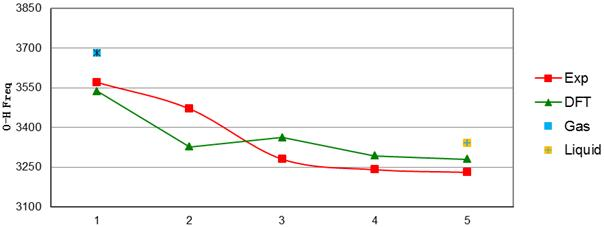Figure 6. Vibrational frequency of (CH3OH)5 in gas phase, a) 5 (cyclic), b) 5ZZ, c) 5ZA, d) 5Z, and inset shows zoomed  O-H Stretching frequencies with number of mode (N in table 6).

It is well-known that gaseous methanol has a higher vibrational frequency for the O-H stretch than liquid methanol (Shimanouchi, 1972). Clearly, even with the small basis set is used in this study, the OH vibrational frequency drops as the number of methanol molecules in the cluster increases. The minimum frequency is essentially attained when there are five methanol's in the cluster, which provides further evidence to support the suggestion that five or six hydrogen-bonded methanol's are sufficient to mimic liquid behavior. Figure 6 shows that results of DFT method become near to experimental values and with increasing of cluster size, frequencies shifted to liquid methanol frequency (3681 and 3340 cm-1 for gas and liquid molecules respectively (Buck and Huisken, 2000)) and another results is in DFT method at the latest OH frequencies are combination vibrations of CH and OH modes in most clusters (for example, 1MCBu, 1,3MCBu1, 1,3MCBu2, 1M2CBu and 1,4MCBu, 1-M-CPe). Figure S2 is showed vibrational spectrometry for pentamer of methanol molecules that is calculated at DFT level of theory.

4. Conclusions

In this study, we have reported that in all of conformers with equal methanol molecule number, the cyclic structure has the most stabilization energy and DFT calculations have the more SE than HF and MP2 levels of theory. The results of this work showed the old n-mers have a slightly greater stability than the even n-mers and the average number of primary hydrogen bonds per methanol molecule increases up to n = 3 but then levels of around one as n approaches 6. The SHB roles prevailing forces opposite steric hindrance in structure of clusters and so caused more stability of them. A BSSE and ZPE correction is noted in the SE calculations. Primary and secondary hydrogen bonding was established by AIM analysis and its effects on SE were investigated. Highervalues obtained by AIM analysis help to establish the nature of hydrogen bonding in the methanol clusters. The maximumvalues are observed in the cyclic structures even though from n=4 onwards they are uniformly around 0.048 a.u.. According to frequencies data, secondary hydrogen bonds lead to decreasing of intensities for inclusive oxygen atoms vibration which this effect have been seen in 1,3MCBu1 and 1,3MCBu2 very clearly.

Supporting information

Supplementary data associated with this article consist of Schematic representation of the most stable geometry for (CH3OH)n, n =1-4 (Figure S1), Vibrational spectroscopy of (CH3OH)5 (Figure S2), Stabilization energy values for three method (Table S1), Effect of BSSE and ZPE corrections on SE (Table S2), Primary hydrogen bonding length, electron density () and laplacian of electron density at BCP in methanol clusters up 4 molecules (Table S3), Geometry properties for methanol structures in DFT level of theory (Table S4), mulliken charge table for oxygen and hydrogen atoms contains in primary hydrogen bonding (Tables S5 and S6 ), and stretching frequencies for O-H in (CH3OH)n, n = 1-4 and its isomers (Table S7) is provided in the supporting information.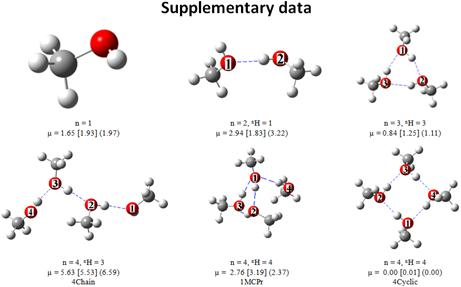Figure S1. Schematic representation of the most stable geometry for (CH3OH)n, n =1-4, along with the number of primary hydrogen bonds (nH) and total dipole moment values in Debye as obtained from DFT calculations using the 6-31G(d) basis set. The values in brackets and parentheses correspond to µ values obtained from MP2 and HF calculations respectively.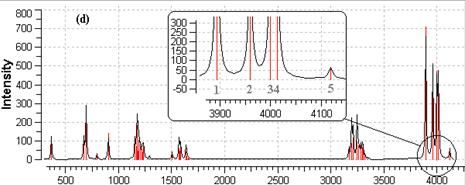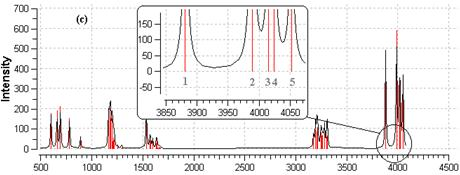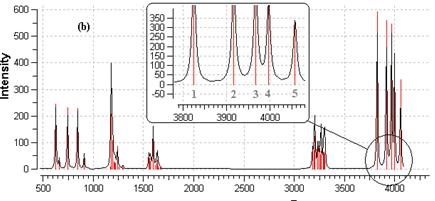Figure S2. Vibrational spectroscopy of (CH3OH)5 in gas phase, a) 5Cyclic, b) 1MCBu, c) 1M2CPr, d)5Chain, and inset shows zoomed O-H Stretching frequencies.

Table S1. Stabilization energy in kcal/mol at the HF, DFT and MP2 methods for (CH3OH)n , n=2-6.

 -SE Structure HF DFT MP2 2 5.5 7.5 4.9 3Cyclic 16.8 24.2 15.3 4Chain 20.3 28 18.7 1MCPr 22.8 33.3 20.8 4Cyclic 28.4 39.9 26.2 5Chain 28.9 40.7 26.3 1M2CPr 30.4 43.7 27.9 1MCBu 33.8 48.6 30.6 5Cyclic 37.1 51.3 34.1 1M3CPr 38 54.6 34.5 1,3MCBu1 39.7 57.2 35.8 1,3MCBu2 40.8 58.1 37.1 1,4MCBu 40.7 58.3 37 1M2CBu 41.6 59.6 37.2 1MCPe 43.1 60.5 38.6 6Boat 45.3 62.8 41.4 6Chair 45.8 62.5 42.2

Table S2. Effect of BSSE and ZPE corrections on SE (kcal/mol) at the DFT/6-31G(d) level for (CH3OH)n, n=2-5.

 -SE Structure BSSE Uncorrected for BSSE and ZPE BSSE corrected ZPE corrected 2 -2.7 7.5 4.7 5.8 3Cyclic -7.2 24.2 17.0 20.0 4Chain -7.8 28 20.2 23.3 1MCPr -11.3 33.3 21.9 27.4 4Cyclic -10.2 39.9 29.6 21.8 5Chain -11.6 40.7 29.1 34.1 1M2CPr -13.4 43.7 30.3 36.5 1MCBu -14.0 48.6 34.6 41.1 5Cyclic -10.9 51.3 40.3 44.4

Table S3. Primary hydrogen bonding length, electron density (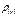) and laplacian of electron density at BCP (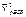) in methanol clusters up to 4 molequels at the DFT/6-31G(d) method.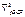is in negative values.

 Structure (H…O)1,2/RHO/ (H…O)2,3/RHO/ (H…O)3,4/RHO/ (H…O)4,1/RHO/ Dimer 1.898/0.032/0.023 3Cyclic 1.828/0.037/0.026 1.845/0.035/0.026 1.825/0.037/0.027 4Chain 1.951/0.037/0.027 1.886/0.044/0.033 1.944/0.039/0.027 1MCPr 1.739/0.045/0.032 1.871/0.033/0.024 1.906a/0.030/0.022 1.902/0.031/0.022 4Cyclic 1.721/0.046/0.033 1.721/0.046/0.033 1.721/0.046/0.033 1.721/0.046/0.033

a for primary hydrogen bonding between (H…O)1,3.

Table S4. Geometry properties for methanol structures in DFT level of theory.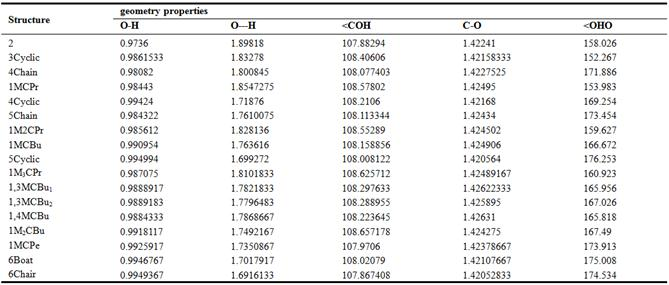Table S5. Mulliken charge for oxygen and hydrogen atoms contains in primary hydrogen bonding up 4 methanol clusters at DFT/6-31G(d) method. Oxygen charge is in negative values and cluster names arrangement by the stability.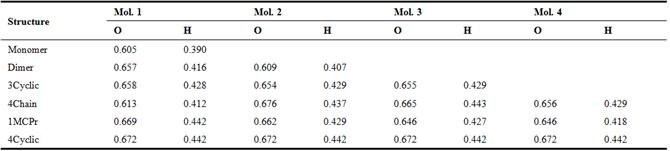Table S6. Mulliken charge for oxygen and hydrogen atoms contains in primary hydrogen bonding from 5 to 6 methanol clusters at DFT/6-31G(d) method. Oxygen charge is in negative values and cluster names arrangement by the stability.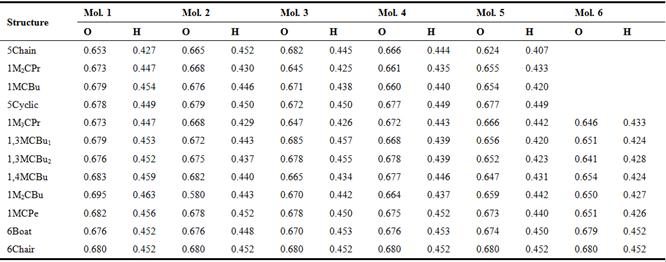Table S7. Stretching frequencies calculated at DFT/6-31G(d) optimized geometry for O-H in (CH3OH)n, n = 1-4 and its isomers. Frequencies are scaled in cm-1 and and IMV (intensity of molecule’s vibration) denotes to O-H intensities at any molecule.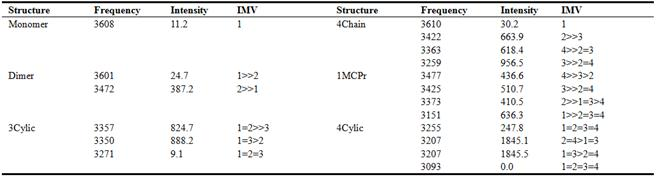Acknowledgements

We gratefully acknowledge the financial support of this work by the Drug Applied Research Center, Tabriz University of Medical Sciences. We are very grateful to Dr. Solimannejad of the Arak University and Dr. Massahi of the Ilam University for helpful discussions.

References

1. Saiful Islam, M., Pethrick, R.A., Pugh, D., (1998) Ab Initio Study of Potassium Ion Clusters of Methanol and Acetonitrile and a Systematic Comparison with Hydrated Clusters, J. Phys. Chem. A, 102, 2201.
2. Choi, J.H, Park, K.W., Park, IS., Nam, W.H., Sung, YE., (2004) Methanol electro-oxidation and direct methanol fuel cell using Pt/Rh and Pt/Ru/Rh alloy catalysts, Electrochim. Acta, 50, 787.
3. Azizi, O., Jafarian, M., Gobal, F., Heli, H., Mahjani, M.G., (2007) The investigation of the kinetics and mechanism of hydrogen evolution reaction on tin, Int. J. Hydrogen Energy, 32, 1755.
4. Sum, A.K., Sandler, S.I., (2000) Ab Initio Calculations of Cooperativity Effects on Clusters of Methanol, Ethanol, 1-Propanol, and Methanethiol, J. Phys. Chem. A, 104, 1121.
5. Boyd, S.L., Boyd, R.J., (2007) A density functional study of methanol clusters, J. Chem. Theory Comput., 3, 54.
6. Haughney, M., Ferrario, M., McDonald, I.R., (1987) Molecular-dynamics simulation of liquid methanol, J. Phys. Chem., 91, 4934.
7. Handgraaf, J.W., Van Erp, T.S., Meijer, E.J., (2003) Ab initio molecular dynamics study of liquid methanol, Chem. Phys. Lett., 367, 617.
8. Morrone, J.A., Tuckerman, M.E., (2002) Ab initio molecular dynamics study of proton mobility in liquid methanol, J. Chem. Phys., 117, 4403.
9. Mejia, S.M., Espinal, J.F., Mondragon, F., (2009) Cooperative effects on the structure and stability of (ethanol)3-water, (methanol)3-water heterotetramers and (ethanol)4, (methanol) 4 tetramers, J. Molecular Structere: Theochem, 901, 186.
10. Garcia-Muruais, A., Cabaleiro-Lago, E.M., Hermida-Ramon, J.M., Rios, M.A., (2000) The study of A(CH3OH)(1-6) (A = Li+, Na+) in the gas phase based on ab initio calculations, analysis of the solvation process, Chem. Phys., 254, 109.
11. Frisch, M.J., Trucks, G.W., Schlegel, H.B., Scuseria, G.E., Robb, M.A., Cheeseman, J.R., Montgomery Jr, J.A., Vreven, T., Kudin, K.N., Burant, J.C., Millam, J.M., Iyengar, S.S., Tomasi, J., Barone, V., Mennucci, B., Cossi, M., Scalmani, G., Rega, N., Petersson, G.A., Nakatsuji, H., Hada, M., Ehara, M., Toyota, K., Fukuda, Hasegawa, R., J., Ishida, M., Nakajima, T., Honda, Y., Kitao, O., Nakai, H., Klene, M., Li, X., Knox, J.E., Hratchian, H.P., Cross, J.B., Bakken, V., Adamo, C., Jaramillo, J., Gomperts, R., Stratmann, R.E., Yazyev, O., Austin, A.J., Cammi, R., Pomelli, C., Ochterski, J.W., Ayala, P.Y., Morokuma, K., Voth, G.A., Salvador, P., Dannenberg, J.J., Zakrzewski, V.G., Dapprich, S., Daniels, A.D., Strain, M.C., Farkas, O., Malick, D.K., Rabuck, A.D., Raghavachari, K., Foresman, J.B., Ortiz, J.V., Cui, Q., Baboul, A.G., Clifford, S., Cioslowski, J., Stefanov, B.B., Liu, G., Liashenko, A., Piskorz, P., Komaromi, I., Martin, R.L., Fox, D.J., Keith, T., Al-Laham, M.A., Peng, C.Y., Nanayakkara, A., Challacombe, M., Gill, P. M.W., Johnson, B., Chen, W., Wong, M.W., Gonzalez, C., Pople, J.A., (1998) Gaussian 03, Revision E.01, Gaussian, Inc., Pittsburgh, PA.
12. Boys, S.F., Berardi, F., (1970) The calculation of small molecular interactions by the differences of separate total energies. Some procedures with reduced errors, Mol. Phys., 19, 553.
13. Bader, R.F.W., Biegler-Konig, F., Schonbohm, J., (2002) AIM2000 Program Package, Ver. 2.0, University of Applied Sciences, Bielefield, Germany.
14. Lovas, F.J., Belov, S.P., Tretyakov, M.Y., Stahl, W., Suenram, R.D., (1995) The a-Type K = 0 Microwave Spectrum of the Methanol Dimer, J. Mol. Spectrosc., 170, 478.
15. Shimanouchi, T., (1972) Tables of Molecular Vibration Frequencies Washington, Vol. 1, 105.
16. Buck, U., Huisken, F., (2000) Infrared spectroscopy of size-selected water and methanol clusters, Chem. Rev., 100, 3863.

 Contents 1. 2. 3. 3.1. 3.2. 3.3. 4.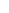600 ATLANTIC AVE, BOSTON,
MA 02210, USA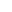+001-6179630233
JournalsJournal ListsAuthor GuidelinesPayment InformationSubmit a ManuscriptOpen Special IssuesJoin as Editorial MembersJoin as ReviewersPrivacy NoticeTerms of ConditionsHelp Center